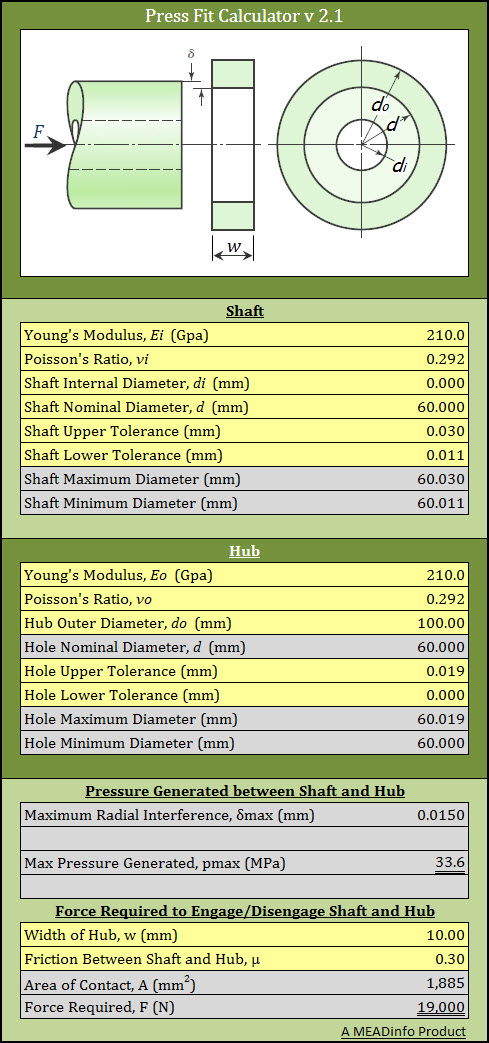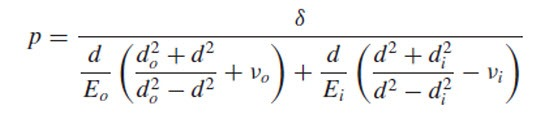Oct 21, 2012

# Press Fit Pressure Calculator – Optimize Your Interference/Transition Fit DesignCalculate pressure generated and force to engage/disengage - H6m6 fit example - Click on the image to download the calculator
Interference and Transition fits can be used effectively connect machine elements. This calculator uses following formula to find the pressure generated with the designed connection.This data then can be used to optimize the design of the hub/shaft, or can be used to find the force to engage and disengage these parts, or can be used to find the maximum torque this connection can transmit without slippage.
Above example shows a transition fit of H6/m6 between shaft and hub.
To find tolerances for different fits refer Tolerance Table for Regularly Used Fits
For calculating minimum force to engage or disengage these parts we can use the following formula
`F = μ . pmax . A`
Where μ is the friction between hub and shaft, A is the area of contact between shaft and hub.
To calculate maximum torque which can be transmitted without slip can be found using following formula
`T = F . d/2`

1.       Minimum force required to engage/disengage as well as maximum (based on the minimum pressure generated)

2.       Added some conditional checks to tell you if a fit is metal-to-metal or a clearance fit, instead of just giving the #VALUE! error.

3.       Added some extra lines to calculate the min/max torque that can be transmitted based on the formula shown on the website